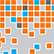# Online Program

Friday, May 31Machine Learning E-Posters, I
Fri, May 31, 9:45 AM - 10:45 AM
Grand Ballroom Foyer

### Comparing Performance of Lasso, Group Lasso, and Linear Regression with Categorical Predictors (306277)

*Yihuan Huang, UCLA

Keywords: Categorical Data, Lasso, Group Lasso, Monte-Carlo Simulation

Machine learning is used frequently to train models and predict outcomes in different scientific areas. Lasso is a method that perform variable selection and regularization, and is often regarded as an advanced version of linear regression. People try to use lasso in the same way as linear regression, assuming they share same properties. For models with categorical predictors, group lasso has been suggested as an alternative to lasso to align with properties from linear regression. The goal of my project is to show that linear regression, lasso, and group lasso have distinct pros and cons and should be treated accordingly. By analyzing wage data with 6 variables with 20 categories total, we determined that lasso predicts better than group lasso which predicts better than linear regression. We also analyzed the effect of choosing different coding strategies on the predicted results. Linear regression is not affected when different coding strategies are chosen. However, using different coding strategies for categorical predictors, lasso builds model with different variable selection. Group lasso fixes the issue with coding strategy, but it can cause overfitting. Using Monte-Carlo simulation, we created a categorical predictor with one dominant category and several non-predictive categories. When there are few non-predictive categories, group lasso is more likely to include the categorical variable with only one dominant category than lasso. Group lasso is less likely to include this categorical variable than lasso when the number of non-predictive categories increases. Researchers primarily focus on the similarity between linear regression and lasso, but pay little attention to their different properties, particularly involving categorical predictors. This project demonstrates that when using lasso, the effect of choosing different coding strategies should be considered and group lasso should be avoided when a dominant category is expected.